Conveniently maps data values (numeric or factor/character) to colours according to a given palette, which can be provided in a variety of formats.

col_numeric(
palette,
domain,
na.color = "#808080",
alpha = FALSE,
reverse = FALSE
)

col_bin(
palette,
domain,
bins = 7,
pretty = TRUE,
na.color = "#808080",
alpha = FALSE,
reverse = FALSE,
right = FALSE
)

col_quantile(
palette,
domain,
n = 4,
probs = seq(0, 1, length.out = n + 1),
na.color = "#808080",
alpha = FALSE,
reverse = FALSE,
right = FALSE
)

col_factor(
palette,
domain,
levels = NULL,
ordered = FALSE,
na.color = "#808080",
alpha = FALSE,
reverse = FALSE
)

## Arguments

palette The colours or colour function that values will be mapped to The possible values that can be mapped. For col_numeric and col_bin, this can be a simple numeric range (e.g. c(0, 100)); col_quantile needs representative numeric data; and col_factor needs categorical data. If NULL, then whenever the resulting colour function is called, the x value will represent the domain. This implies that if the function is invoked multiple times, the encoding between values and colours may not be consistent; if consistency is needed, you must provide a non-NULL domain. The colour to return for NA values. Note that na.color = NA is valid. Whether alpha channels should be respected or ignored. If TRUE then colors without explicit alpha information will be treated as fully opaque. Whether the colors (or color function) in palette should be used in reverse order. For example, if the default order of a palette goes from blue to green, then reverse = TRUE will result in the colors going from green to blue. Either a numeric vector of two or more unique cut points or a single number (greater than or equal to 2) giving the number of intervals into which the domain values are to be cut. Whether to use the function pretty() to generate the bins when the argument bins is a single number. When pretty = TRUE, the actual number of bins may not be the number of bins you specified. When pretty = FALSE, seq() is used to generate the bins and the breaks may not be "pretty". parameter supplied to base::cut(). See Details Number of equal-size quantiles desired. For more precise control, use the probs argument instead. See stats::quantile(). If provided, the n argument is ignored. An alternate way of specifying levels; if specified, domain is ignored If TRUE and domain needs to be coerced to a factor, treat it as already in the correct order

## Value

A function that takes a single parameter x; when called with a vector of numbers (except for col_factor, which expects factors/characters), #RRGGBB colour strings are returned (unless alpha = TRUE in which case #RRGGBBAA may also be possible).

## Details

col_numeric is a simple linear mapping from continuous numeric data to an interpolated palette.

col_bin also maps continuous numeric data, but performs binning based on value (see the base::cut() function). col_bin defaults for the cut function are include.lowest = TRUE and right = FALSE.

col_quantile similarly bins numeric data, but via the stats::quantile() function.

col_factor maps factors to colours. If the palette is discrete and has a different number of colours than the number of factors, interpolation is used.

The palette argument can be any of the following:

1. A character vector of RGB or named colours. Examples: palette(), c("#000000", "#0000FF", "#FFFFFF"), topo.colors(10)

2. The name of an RColorBrewer palette, e.g. "BuPu" or "Greens".

3. The full name of a viridis palette: "viridis", "magma", "inferno", or "plasma".

4. A function that receives a single value between 0 and 1 and returns a colour. Examples: colorRamp(c("#000000", "#FFFFFF"), interpolate="spline").

## Examples

pal <- col_bin("Greens", domain = 0:100)
show_col(pal(sort(runif(10, 60, 100))))# Exponential distribution, mapped continuously
show_col(col_numeric("Blues", domain = NULL)(sort(rexp(16))))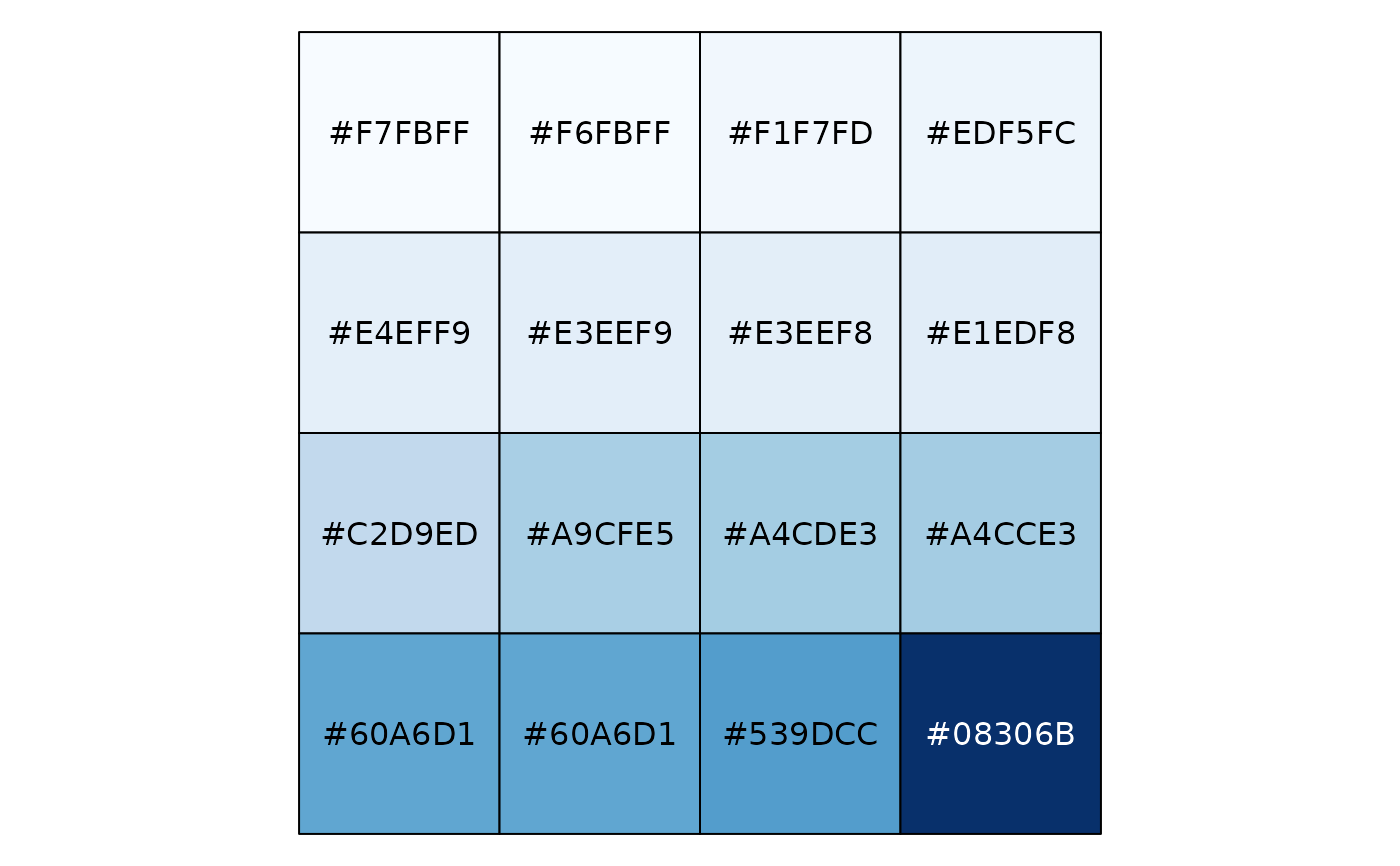# Exponential distribution, mapped by interval
show_col(col_bin("Blues", domain = NULL, bins = 4)(sort(rexp(16))))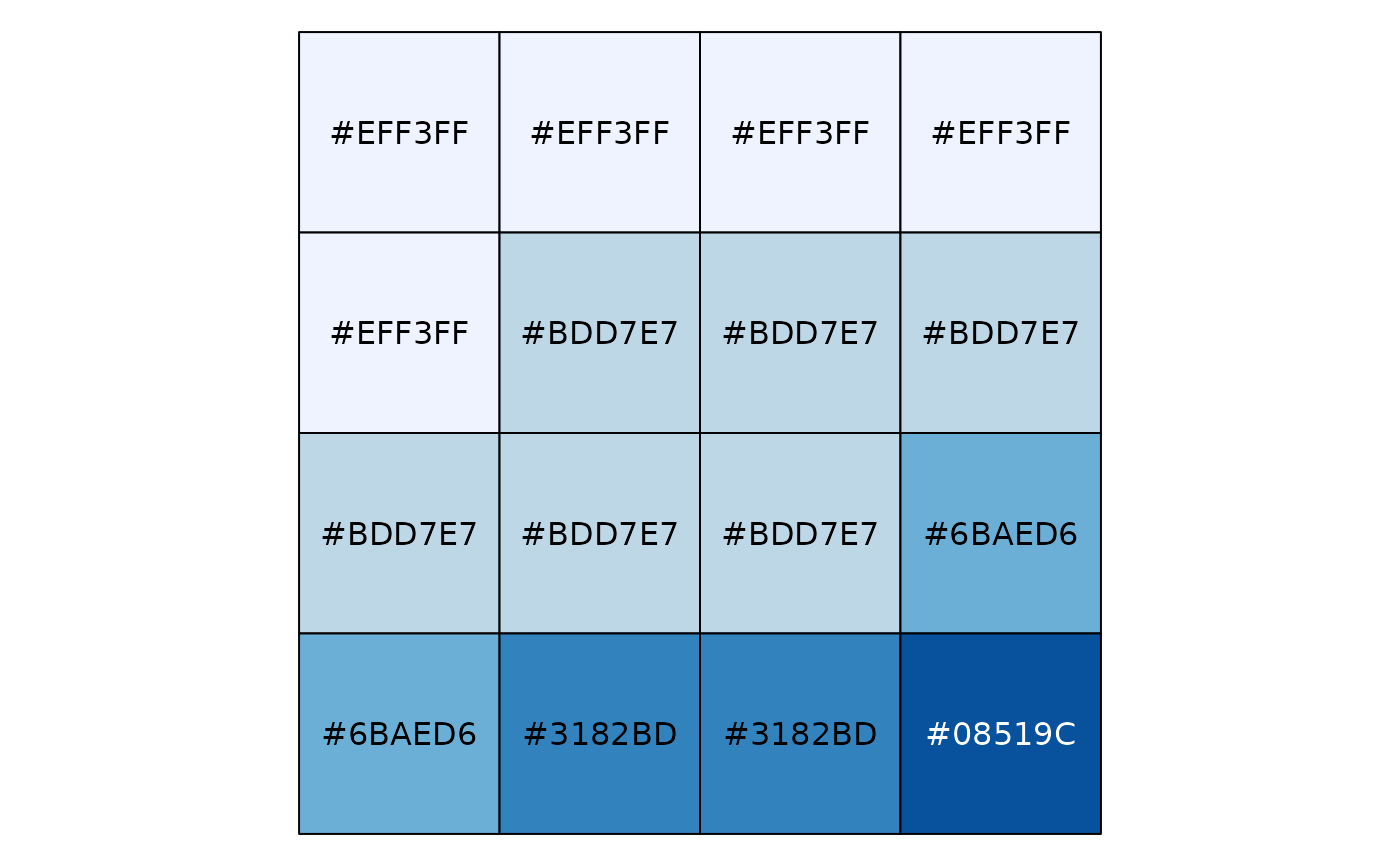# Exponential distribution, mapped by quantile
show_col(col_quantile("Blues", domain = NULL)(sort(rexp(16))))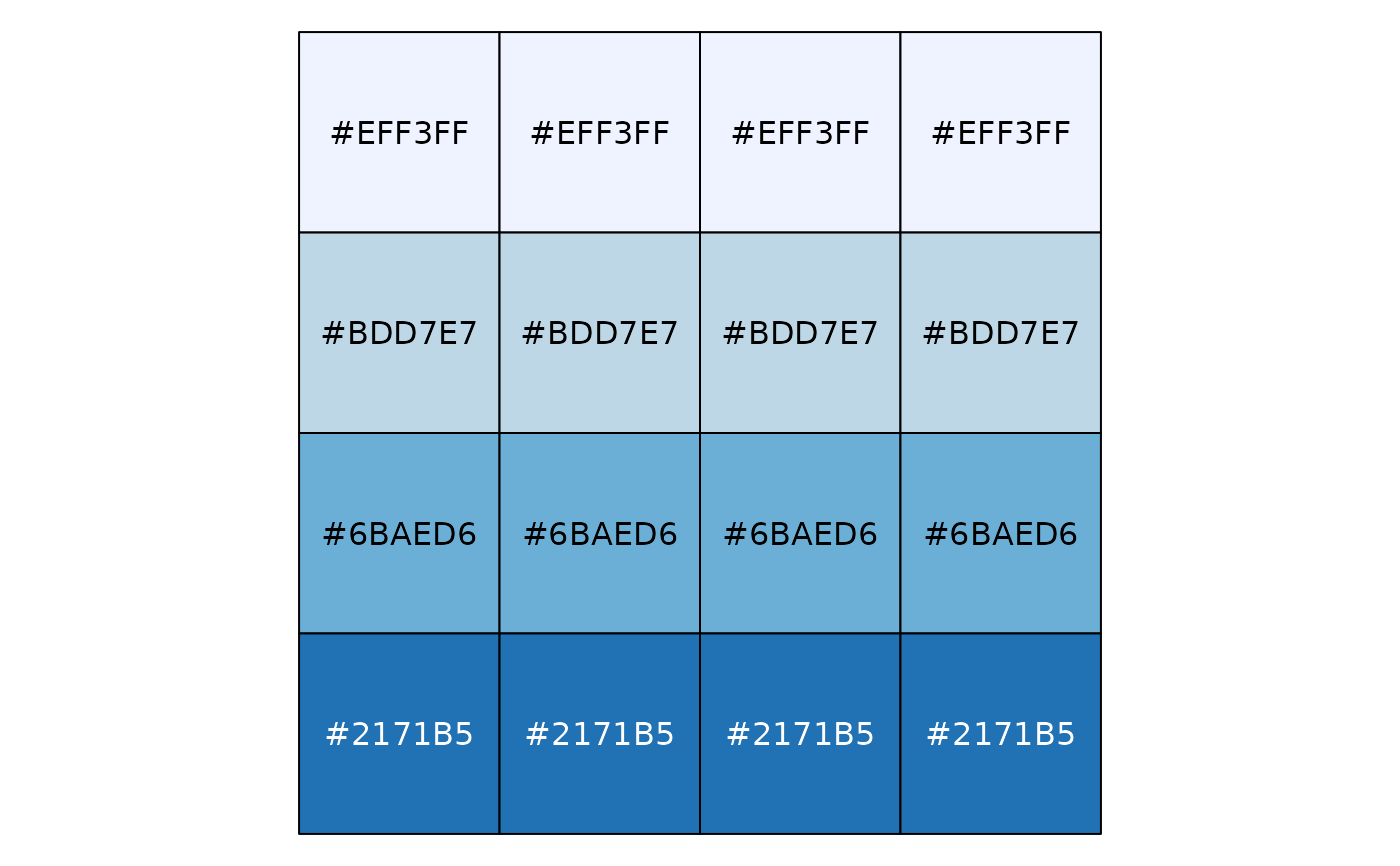# Categorical data; by default, the values being coloured span the gamut...
show_col(col_factor("RdYlBu", domain = NULL)(LETTERS[1:5]))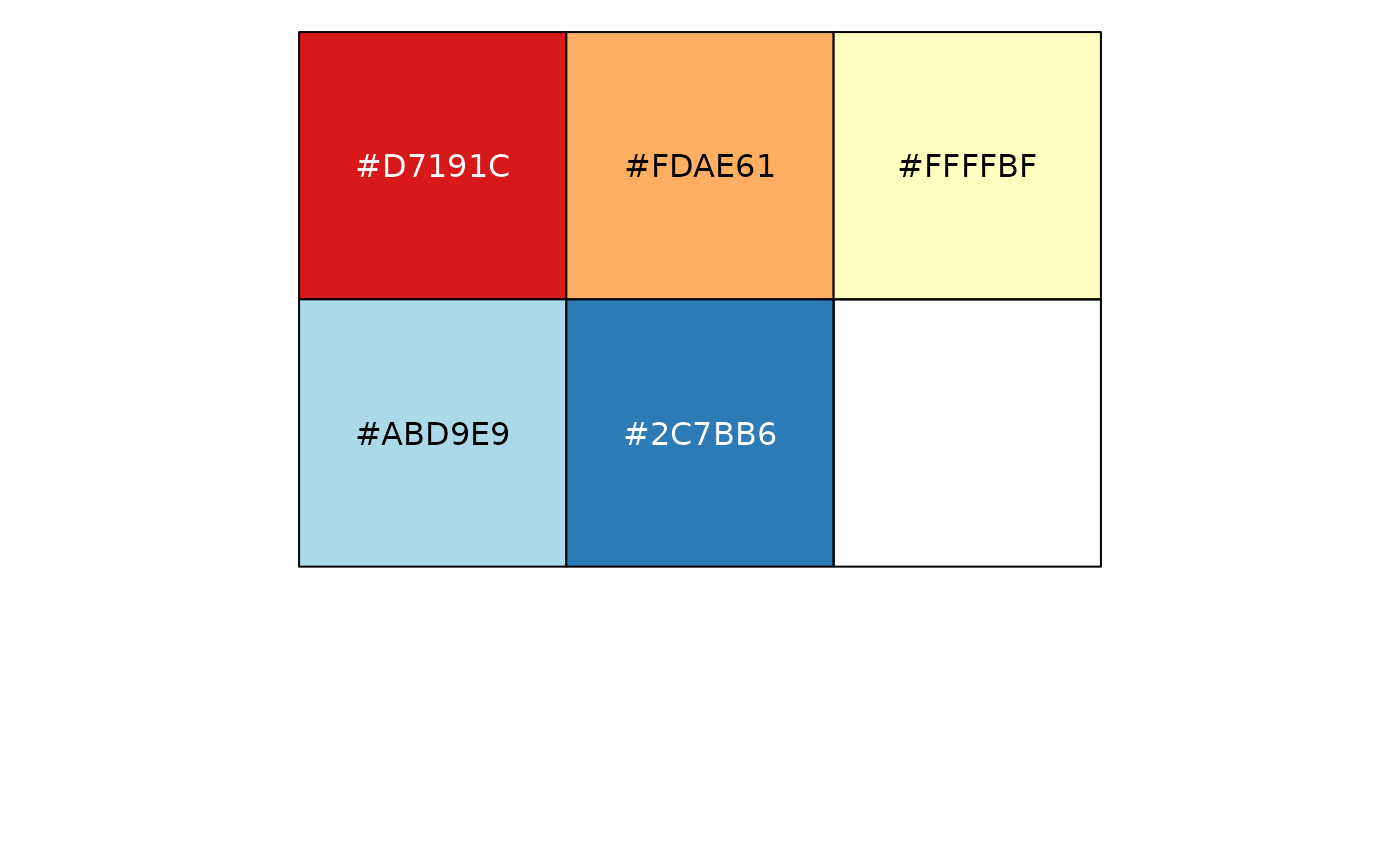# ...unless the data is a factor, without droplevels...
show_col(col_factor("RdYlBu", domain = NULL)(factor(LETTERS[1:5], levels=LETTERS)))
#> Warning: n too large, allowed maximum for palette RdYlBu is 11
#> Returning the palette you asked for with that many colors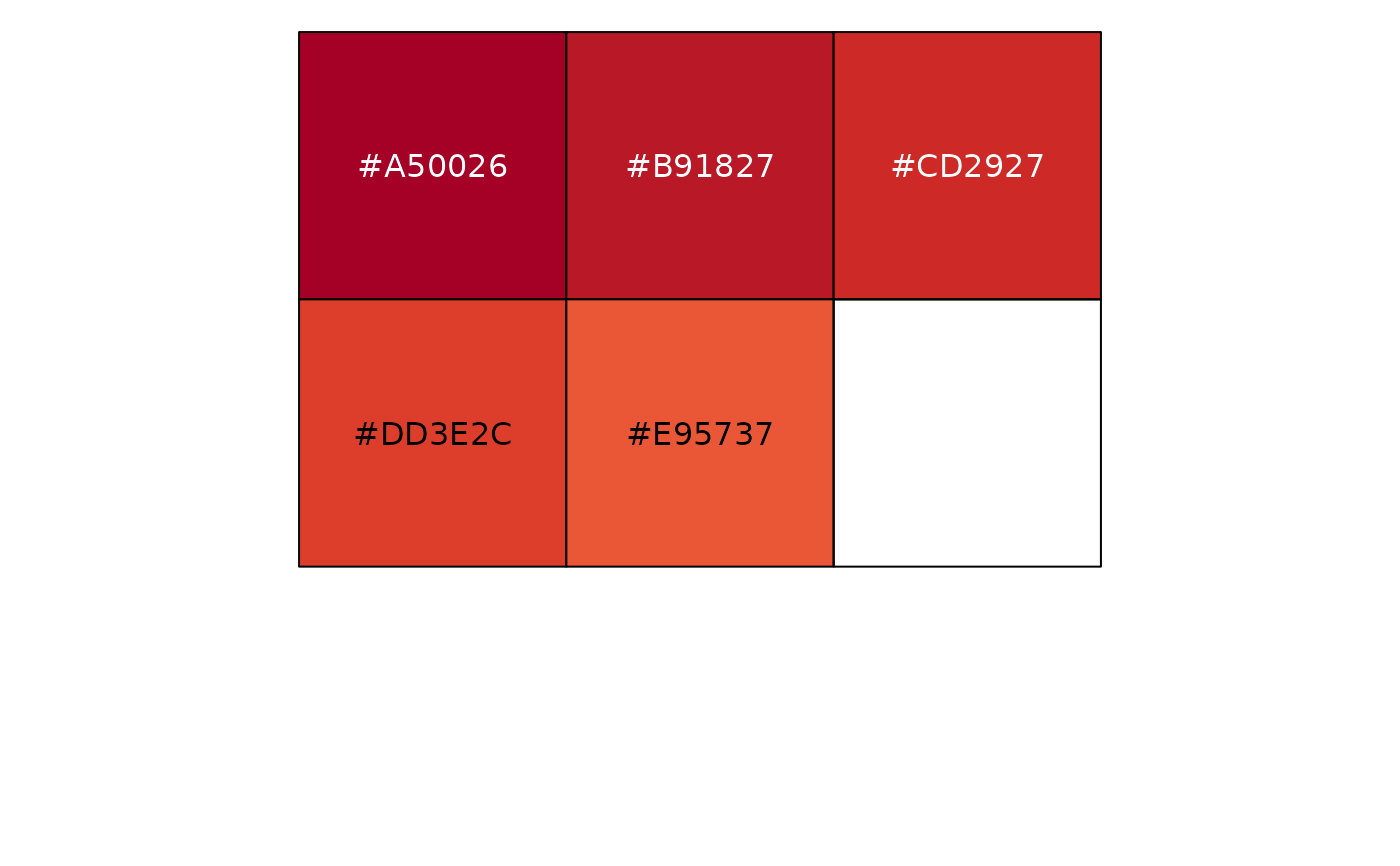# ...or the domain is stated explicitly.
show_col(col_factor("RdYlBu", levels = LETTERS)(LETTERS[1:5]))
#> Warning: n too large, allowed maximum for palette RdYlBu is 11
#> Returning the palette you asked for with that many colors